Login or Create an Account to view question mark schemes, comment, and download a PDF of this test
Question 1[1 Mark]
Choose which of the following describes a key difference between an array and a linked list.
(a).
An array is a non-linear data structure, while a linked list is a linear data structure.
(b).
In an array, elements are stored in non-contiguous memory locations, while in a linked list, elements are stored in contiguous memory locations.
(c).
An array is a linear data structure, while a linked list is a non-linear data structure.
(d).
In an array, elements are stored in contiguous memory locations, while in a linked list, elements are stored in non-contiguous memory locations.
Question 2[1 Mark]
Select the description below which does NOT accurately characterise how computational recursion works.
(a).
Recursion is the repeated application of a self-referencing function or procedure.
(b).
Recursion decomposes a problem into increasingly smaller instances of the same problem.
(c).
Recursion must eventually reach a base case in order to stop making further recursive calls.
(d).
Recursion must aggregate results returned from recursive calls to form a solution.
Question 3[1 Mark]
Assuming the typical binary search tree structure, select the procedure which logically represents an in-order binary search tree traversal.
(a).
method INORDER(tree) INORDER(right-tree) OUTPUT current node INORDER(left-tree) end method
(b).
method INORDER(tree) INORDER(left-tree) INORDER(right-tree) OUTPUT current node end method
(c).
method INORDER(tree) OUTPUT current node INORDER(right-tree) INORDER(left-tree) end method
(d).
method INORDER(tree) INORDER(left-tree) OUTPUT current node INORDER(right-tree) end method
Question 4[4 Marks]
Memory management is an important function of modern Operating Systems (OS). Outline one benefit and one disadvantage of OS memory management.
Question 5[7 Marks]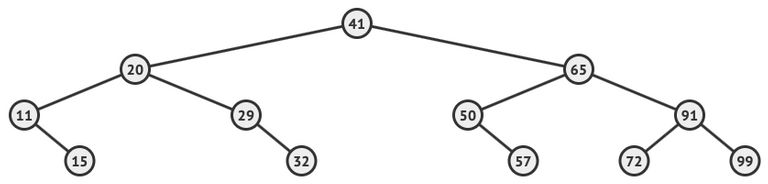In consideration of the binary tree shown above
(a).
State the value contained in each of the following:
(i).
The root node

(ii).
The last node visited by an pre-order traversal

(iii).
The leaves of the largest possible left subtree

(iv).
The right child of the sibling of the node containing 65

(b).
Draw a resulting tree if the node containing the value 65 were removed

Question 6[11 Marks]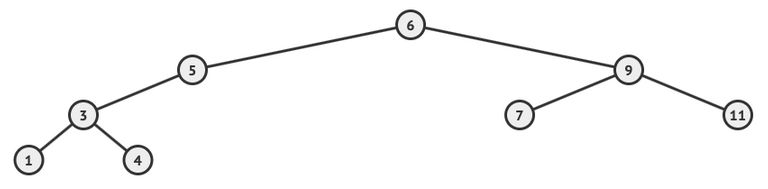(a).
With respect to the binary search tree above, list all the nodes values according to the order in which they are visited by the following tree traversals.
(i).
In-order

(ii).
Pre-order

(iii).
Post-order

(b).
Describe how the post-order tree traversal algorithm works.

(c).
In contrast to arrays, outline two benefits of using binary search trees.

(d).
In contrast to a balanced binary search tree, identify one disadvantage of an unbalanced binary search tree;

Question 7[3 Marks]
Describe why polling the status of an external device may be more efficient than allowing the same device to interrupt the CPU.
Question 8[1 Mark]
Select the boolean function equivalent to the logic gate configuration represented below.(a).
Z = X NOR Y
(b).
Z = X XOR Y
(c).
Z = X AND Y
(d).
Z = X NAND Y
Question 9[6 Marks]
Consider the following boolean expression:
(A AND NOT B) OR (B AND NOT A)
(a).
Construct a truth table for the boolean expression above.

(b).
Hence or otherwise, state the simple boolean expression composed of only one logical operator which is logically equivalent to the boolean expression above.

Question 10[4 Marks]
Construct both a logic diagram and truth table for the following expression:
NOT A OR A AND B
Question 11[2 Marks]
Construct the logic circuit for the following Boolean expression.
X = NOT P OR Q AND P
Question 12[1 Mark]
Name the single gate logically equivalent to the configuration represented below.Question 13[4 Marks]
(a).
Construct a truth table for the logic diagram represented below.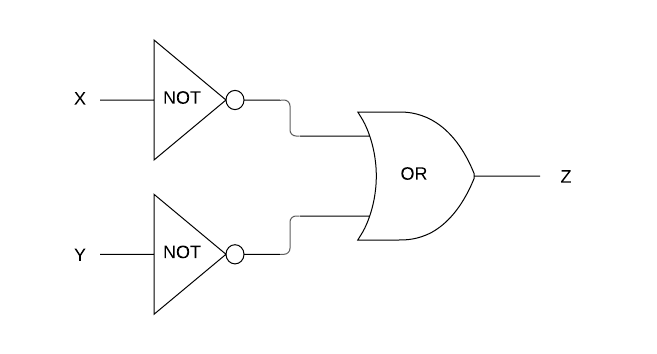(b).
What single gate is logically equivalent to the configuration represented in part (a)?

(c).
If, in part (a), the OR gate was replaced by an AND gate to produce the logic diagram below, what single gate would it then become logically equivalent to?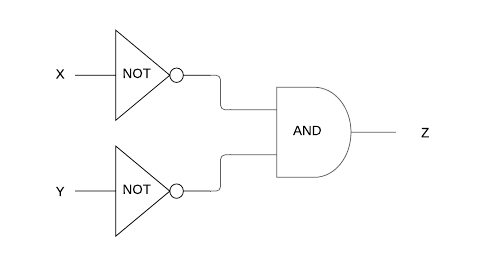Question 14[4 Marks]
Construct both a logic diagram and truth table for the following expression:
A AND (A OR NOT B)
Question 15[12 Marks]
At the moment marathon runners cross the finishing line of a race, their names are added to a stack called RUNNERS.
For example, a diagram of RUNNERS below indicates “Ellie” has finished first and “Jessie” last. “Jessie” is at the top of the RUNNERS stack.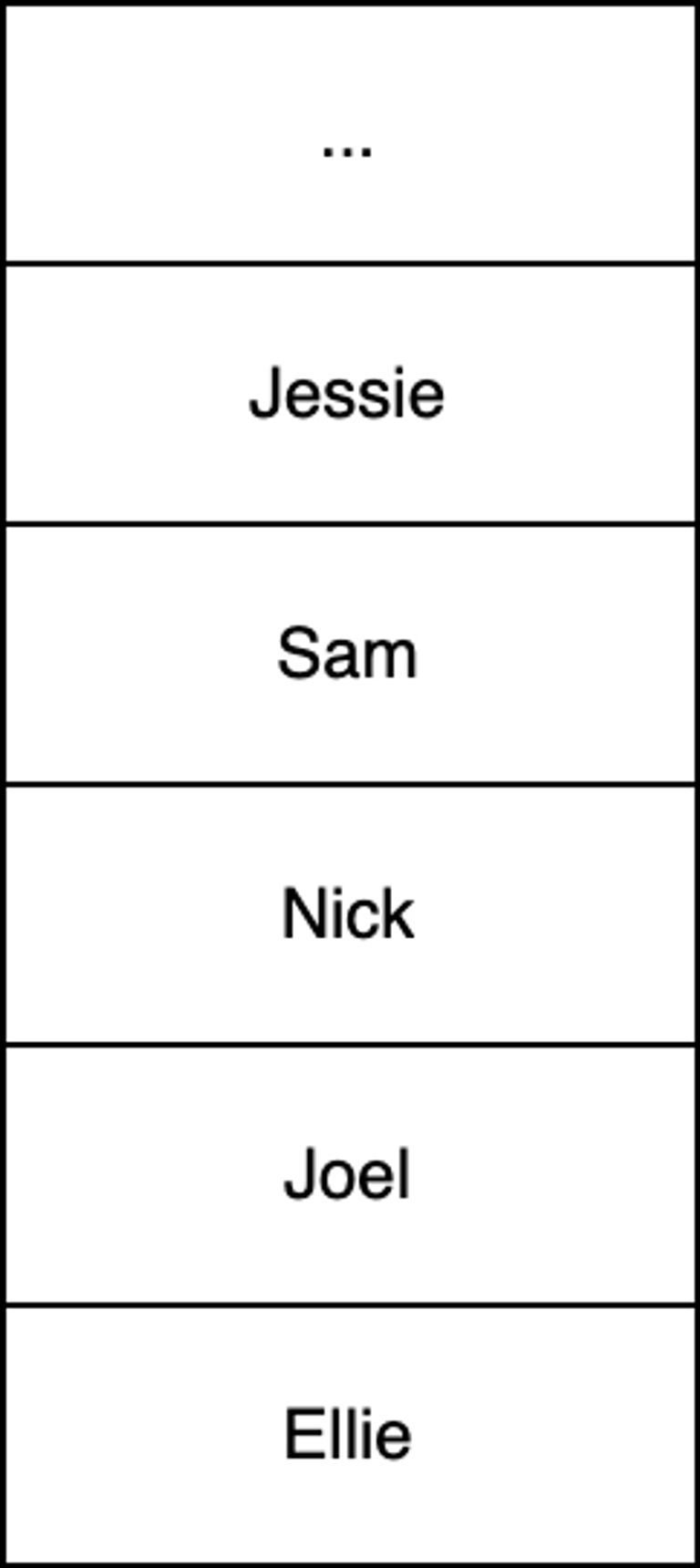(a).
Using pseudocode, construct an efficient procedure that will search the stack RUNNERS for a name given via standard input. Once the given name is found, output the finishing place of the runner specified as a number. If a name is not found then the procedure should output the message "Runner not found". Assume all names are unique, the number of elements in RUNNERS is unknown and RUNNERS may be emptied during the search process.

For example, given an input string of "Joel" the procedure will output the number 2 to indicate Joel finished in 2nd place.
(b).
Outline why the use of a queue would make this algorithm simpler and more efficient than using a stack.

In the same way as RUNNERS saves each runner's name, another stack called TIMES saves each runner's finishing time as they cross the finish line.
(c).
The results of a particular competition where 101 runners successfully finish a race are held in RUNNERS and TIMES. Given an empty 101x2 (row x column) 2D array called RESULTS, write pseudocode such that the first column contains the names of all those runners who finished the race and the second column contains their respective times in ascending rank order.

For example, RESULTS should contain the time of the runner who finished in 94th place, that is, having only ran faster than seven people (eighth last).
(d).
Using RESULTS, state the single line of pseudocode that represents the name of the runner with the median finishing time.

Question 16[4 Marks]
2D array transposition reflects array values across the top left to bottom right diagonal such that the row and column indices of each value in the array are swapped.
Construct an algorithm to efficiently transpose a 2D array called NUMBERS of size 3x3. NUMBERS must be transposed in-place, that is, the values in the original array must be replaced by the transposed values (a new array should not be created).
For example, consider the following 2D array: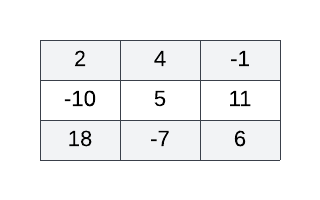The correctly functioning algorithm applied to the 2D array above yields the following transposed 2D array: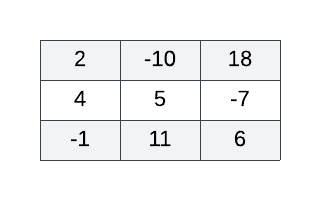Question 17[1 Mark]
Which of the following gates implement the equivalent logic to the configuration represented below?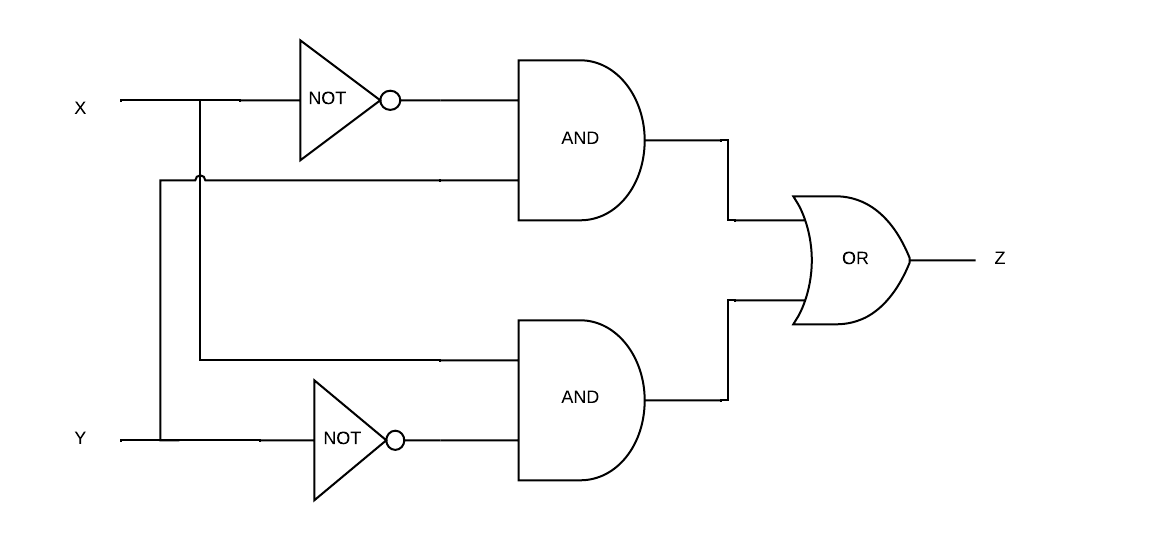(a).
NOR
(b).
XOR
(c).
XNOR
(d).
NAND
Question 18[4 Marks]
Write an algorithm to output every number greater than 7 in a 4 x 3 (row by column) 2D array of numbers. Numbers may be output in any order.
For example, the correctly functioning algorithm applied to the following 2D array yields 11 and 18: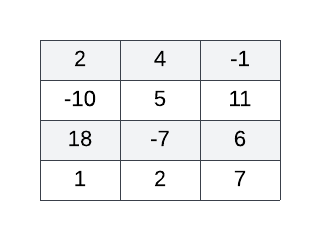Question 19[5 Marks]
Given a 2D array called SCORES[row index][column index] of size 4x3 (rows x columns) where each row contains the game scores for a different computer game, construct an algorithm to output a new 1D array called TOTALS containing the total score of each computer game, that is, the sum of each row in SCORES (in row order).
For example, consider the following 2D array: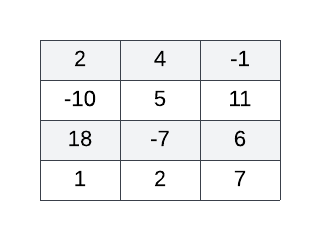The correctly functioning algorithm applied to the 2D array above yields the following 1D array: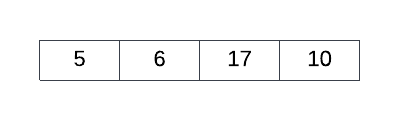Question 20[5 Marks]
Given a 2D array called ARR of size 4x3 and an empty 1D array called BIGGEST of size 4, construct an algorithm to sequentially fill BIGGEST with the biggest number in each ARR row such that the index of each number in BIGGEST is the same as its original ARR row index. The more positive or less negative a number, the bigger it is - for example, 7, 0 and -2 are all considered bigger than -10. ARR may contain one or more negative numbers. For example, consider the following 2D array:The correctly functioning algorithm applied to the 2D array above yields the following 1D array: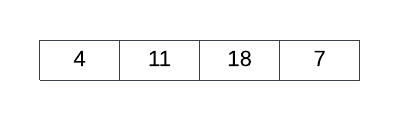Question 21[14 Marks]
Consider the following diagram representing seats in a movie theater where each seat is specified by a row number ranging from 1 to 7 (inclusive) and a column number ranging from 1 to 10 (inclusive). The theater's ticketing system has already allocated Andrew ("A"), Sally ("S") and Rufus ("R") seats for an upcoming screening.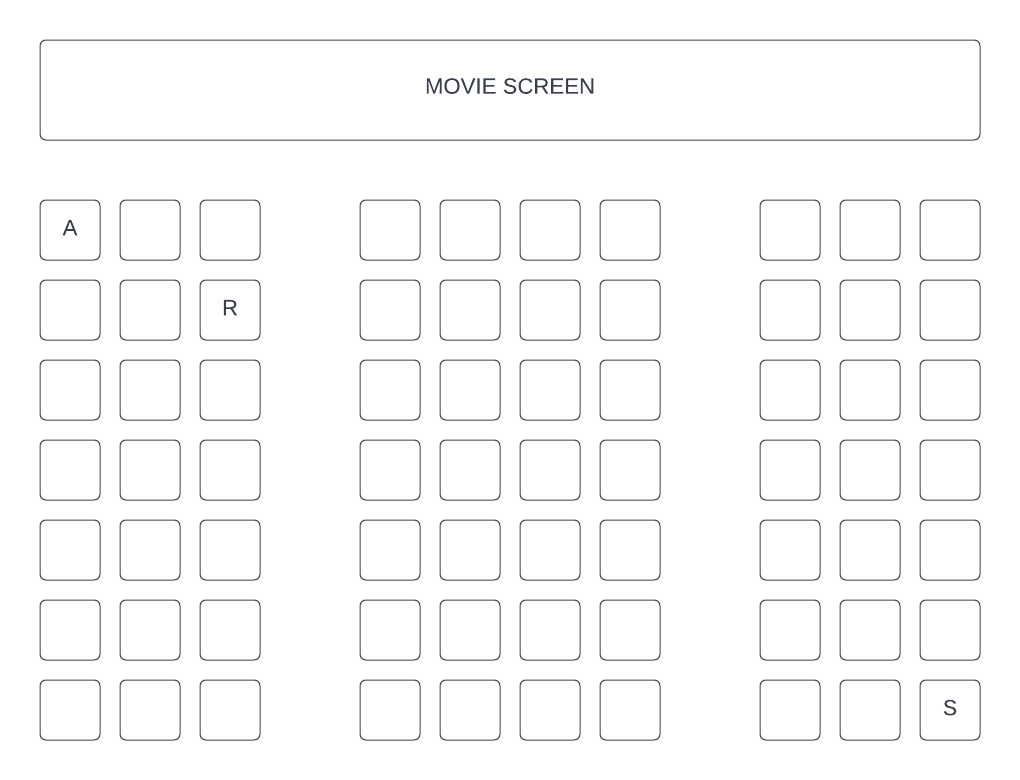The ticketing system stores ticketing information in a collection called TICKETS. TICKETS is composed of nodes where values alternate between integers and strings - each integer has encoded seating information for the customer whose name is held as a string in the next node. The first two digits and the last two digits of each integer specify the row number and column number of a seat respectively. For example, Rufus has a ticket for the third seat across in the second row. TICKETS contains only those customers with a ticket and all ticket holders have exactly one allocated seat each. In TICKETS, it can be assume that a customer name will always follow their seating number. Therefore, customer seating information is always held in sequentially linked pairs - for example: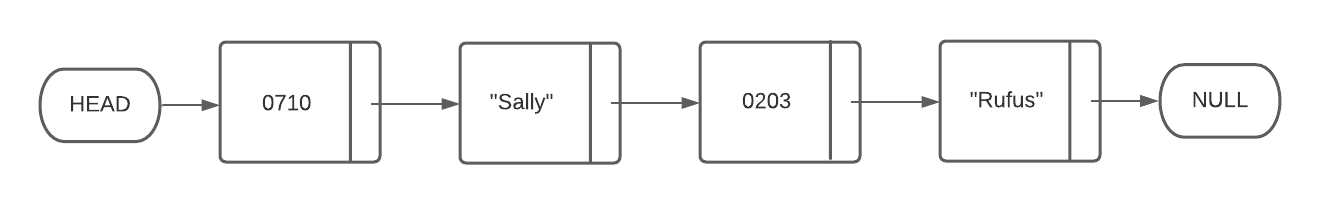(a).
Construct an algorithm to only output all ticket holder names held in TICKETS.

(b).
Describe one problem with storing ticketing information in this way and explain why a 2D array would be more appropriate.

(c).
Given a 7x10 2D array called TIX, construct an algorithm to parse ticketing information from the collection TICKETS into TIX such that ticket holder names are copied to a TIX location representative of their seat location. For example, TIX, TIX, and TIX should hold the names "Andrew", "Rufas" and "Sally" respectively. Unassigned seats should be indicated in TIX by the string "Empty".

Question 22[10 Marks]
(a).
Sketch a doubly linked list holding the same words in the same alphabetical order as those in the array below.

["Apple","Lemon","Peach"]
(b).

(c).
In contrast to singly linked list, describe why using a doubly linked list to implement a stack or queue data structure is unnecessary.

Question 23[6 Marks]
(a).
State what is meant by the term ‘dynamic data structure’

Consider the following circularly linked list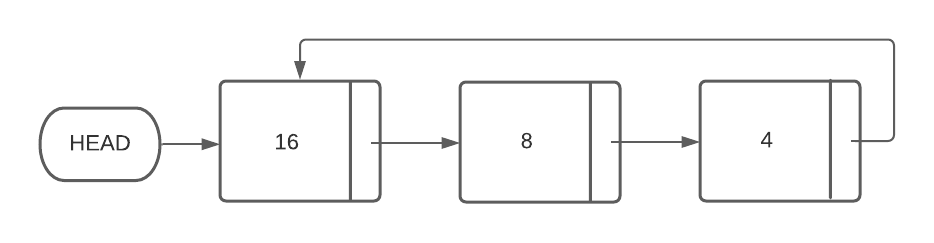(b).
Given a new node containing the number 7 (called NEW_NODE) and a pointer (called PREV_NODE) which points to the node containing the number 8, describe how the new node should be added to the linked list in such a way that the circularly linked list remains both properly formed and sorted.

(c).
In contrast to the process described in part b), outline why it would be less efficient to add the value 32 to a static array containing [16, 8, 4] in such as way as to preserve both existing values and ascending order.

Question 24[4 Marks]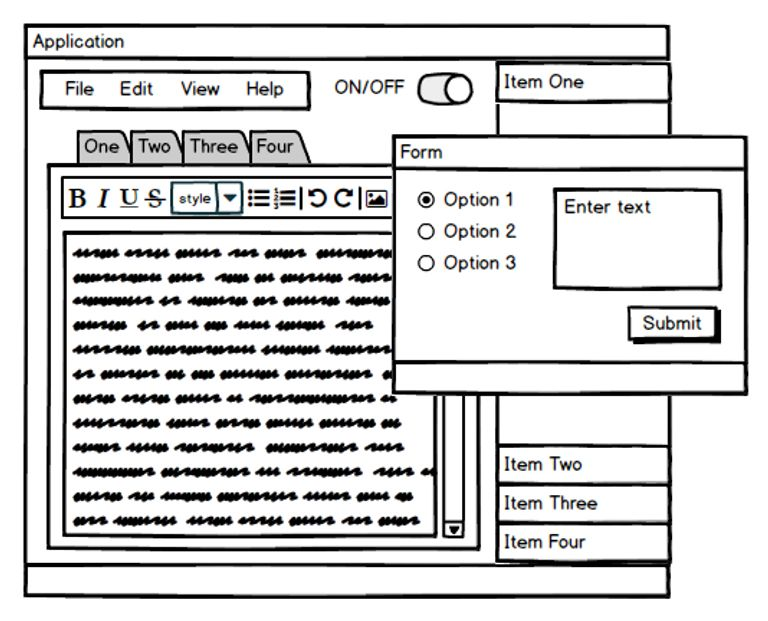Identify five common Graphical User Interface (GUI) features of the application wireframe shown
Question 25[5 Marks]
Consider the following array of four Cartesian co-ordinates called POINTS.
POINTS = [(3,7),(5,11),(1,2),(4,9)]
(a).
Write pseudocode to access the first element in the array.

Consider the following pseudocode which draws a path connecting each point contained in the array POINTS.
drawLine((3,7),(5,11)) drawLine((5,11),(1,2)) drawLine((1,2),(4,9))
(b).
Rewrite the pseudocode above to achieve the same functionality both iteratively and more generally, such that POINTS may contain any four coordinates, each of the form (a, b).

(c).
A particular location at coordinate (7, 7) has been flagged as a private area. Augment your iterative solution from part (b) to always exclude coordinate (7, 7) and at the same time ensure any resultant path is continuous. The coordinate (7, 7) may occur in POINTS more than once.

Question 26[11 Marks]
(a).
Consider the array implementation of a circular queue illustrated below. Each element in the queue is characterised by both an index and value, indicating the underlying array index and element value respectively.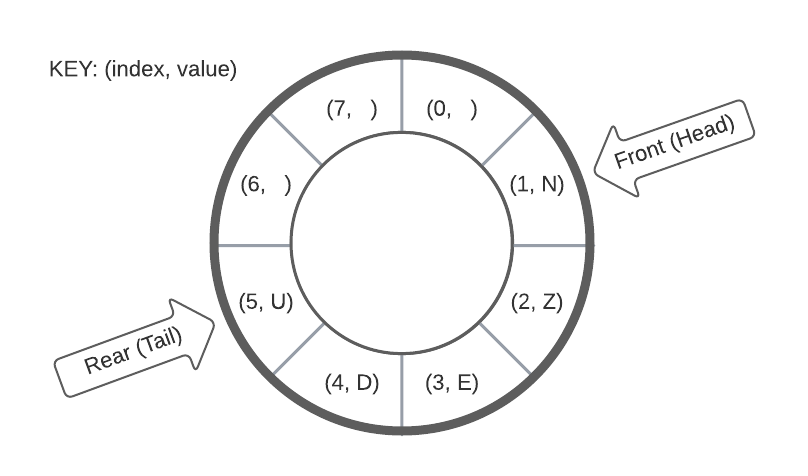(i).
By manipulating the circular queue represented above, draw the result of enqueuing letters "C", "A" & "T" (in their provided written order from left to right i.e. x3 separate enqueue operations) followed by one dequeue operation.

(ii).
Given an array implementation of the circular queue above, state the condition upon which it would be identified as FULL.

(b).
Describe the key functionality of a circular queue.

(c).
Compare and contrast circular queues with linear queues.

Question 27[1 Mark]
A circular queue implementation uses an array of length SIZE to hold queue items as well as two variables called REAR and FRONT to hold the current index positions of the rear (tail) and front (head) of the queue respectively. Enqueue and dequeue functions increment REAR and FRONT respectively. State the conditional statement which would, upon evaluating to True, identify the queue as full.
Question 28[1 Mark]
A circular queue implementation uses an array of length SIZE to hold queue items as well as two variables called REAR and FRONT to hold the current index positions of the rear (tail) and front (head) of the queue respectively. Enqueue and dequeue increment REAR and FRONT respectively. Select the conditional statement below which would, upon evaluating to True, identify the queue as full.
(a).
REAR = (FRONT + 1) % SIZE
(b).
FRONT = (REAR + 1) % SIZE
(c).
REAR + 1 = FRONT % SIZE
(d).
FRONT + 1 = REAR % SIZE
Question 29[1 Mark]
A circular queue implementation uses an array of length SIZE to hold queue items as well as two variables called REAR and FRONT to hold the current index positions of the rear (tail) and front (head) of the queue respectively. Enqueue and dequeue increment REAR and FRONT respectively. Select the conditional statement below which would, upon evaluating to True, identify that the queue only has one element remaining.
(a).
REAR + 1 = FRONT
(b).
REAR = (FRONT + 1) % SIZE
(c).
FRONT = (REAR + 1) % SIZE
(d).
FRONT = REAR
Question 30[1 Mark]
State the advantage of a Circular Queue over a Linear Queue
Question 31[10 Marks]
A busy cafe has decided to streamline their business by implementing a software assisted ordering system. The new system will record customer orders at the front counter whereupon they are automatically added to a queue of orders accessed by a barista working at the back of the cafe to fulfill orders uninterrupted.
(a).
State one reason why a queue would be better suited to holding coffee orders than a stack.

(b).
The new systems holds the price for each type of coffee in an array called ITEMS as follows:

+--------------+--------------+ | Espresso | 1.20 | | Latte | 2.50 | | Cappuccino | 3.70 | +--------------+--------------+
Coffee orders are held in a queue called ORDERS where each order is a single integer indicating the type of coffee to be made by their respective row index in ITEMS array.
Additionally, a 3x3 2D array called SALES is initially defined as follows:
+--------------+--------------+--------------+ | Espresso | 0 | 0 | | Latte | 0 | 0 | | Cappuccino | 0 | 0 | +--------------+--------------+--------------+
Given ITEMS, ORDERS and SALES, write pseudocode such that column one and column two contain the total number of orders and total income for each coffee type respectively.
For example, the following ORDERS queue:
+-------+-------+-------+-------+-------+ 1 0 1 2 0 +-------+-------+-------+-------+-------+
would result in the following SALES array:
+--------------+--------------+--------------+ | Espresso | 2 | 2.40 | | Latte | 2 | 5.00 | | Cappuccino | 1 | 3.70 | +--------------+--------------+--------------+
(c).
The method rowswap(ARR, ROWA, ROWB) acts on the array ARR provided to swap the row at index ROWA with the row at index ROWB.

Without using loops, write pseudocode to sort the rows of SALES from lowest to highest total income. The row for the coffee with the highest total income should be at row index 2.
Question 32[1 Mark]
Name the single gate logically equivalent to the configuration represented below.Question 33[1 Mark]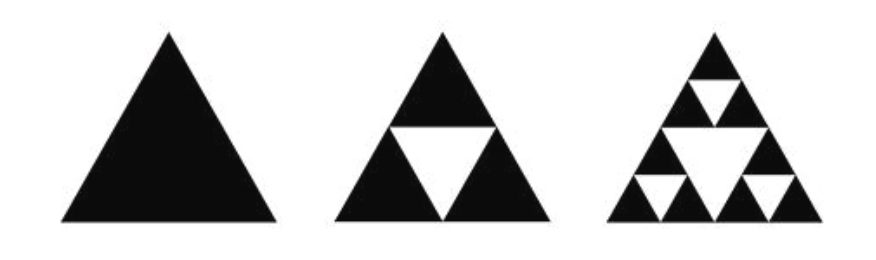Select the concept below which best relates to the abstract pattern above.
(a).
Binary tree traversal
(b).
Recursion
(c).
Fibonacci sequence
(d).
Iteration
Question 34[1 Mark]
Choose the one option below which is not a resource that needs to be managed by the operating system.
(a).
Primary memory
(b).
Kernel
(c).
Screen resolution
(d).
Disk space
Question 35[3 Marks]
Outline the function of the address bus and state one of its roles in the machine execution cycle.
Question 36[1 Mark]
Choose the number of different integers that may be represented using 2 bytes of memory.
(a).
$2^2$
(b).
$2^8$
(c).
$2^{{16}}$
(d).
$2^{{256}}$
Question 37[1 Mark]
Convert the binary number 01011100 held in computer memory to a hexadecimal (base 16) number to be displayed on screen.
Question 38[4 Marks]
Use collection access methods to construct an algorithm in pseudocode which will iterate through a collection of numbers called NUMBERS and count how many numbers it contains that are both even and greater than 7. Output the final count.
Question 39[4 Marks]
Distinguish between Read Only Memory (ROM) and Random Access Memory (RAM).
Question 40[4 Marks]
Contrast the use of array and linked-list data structures.
Question 41[11 Marks]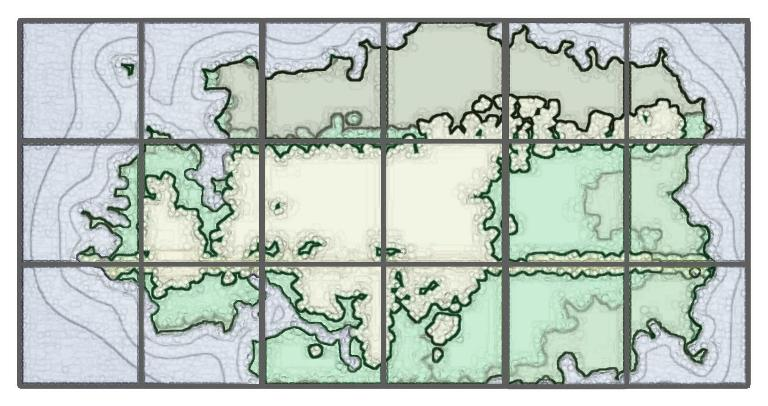Land surveyors use geographic data to determine suitability of grazing land for cows. In this scenario, geographic data is kept in 2D arrays where each element corresponds to an area on the 3x6 (row x column) map grid above. A 2D array called SLOPE, shown below, holds slope data. SLOPE array element contains a number between 0 (inclusive) and 1 (exclusive), approximating the average gradient magnitude for each corresponding section of land.
+-----+-----+-----+-----+-----+-----+ | 0.6 | 0.5 | 0.7 | 0.5 | 0.4 | 0.6 | | 0.7 | 0.3 | 0.2 | 0.2 | 0.3 | 0.2 | | 0.4 | 0.3 | 0.2 | 0.1 | 0.5 | 0.6 | +-----+-----+-----+-----+-----+-----+
Another 2D array called GRASS, shown below, holds grass quality data. GRASS array elements contains a number between 0 and 10 inclusive, approximating the average grass quality of each corresponding section of land where 10 is the best quality.
+-----+-----+-----+-----+-----+-----+ | 9.5 | 8.2 | 8.1 | 9.3 | 7.5 | 8.8 | | 9.1 | 5.1 | 2.7 | 1.2 | 7.2 | 8.3 | | 7.6 | 8.4 | 7.1 | 6.9 | 7.4 | 9.1 | +-----+-----+-----+-----+-----+-----+
Cows do best on flat land with high quality grass. Experts have decided that suitable locations must have both a gradient which is less than 0.4 and a grass quality rating above 7.
(a).
With respect to the data in 2D arrays SLOPE and GRASS, state whether the map location [2,3] is referring to a suitable grazing area and justify your reasons.

(b).
Write a function in pseudocode called isSuitableArea(ROW, COLUMN) which accepts two arguments, a row index called ROW and column index called COLUMN, then returns a boolean indicating whether or not the area specified by ROW & COLUMN is suitable for cattle grazing.

(c).
Using isSuitableArea(ROW, COLUMN), write a function in pseudocode called isSuitableRegion() which returns a boolean indicating if the region described by SLOPE and GRASS data is overall suitable for grazing, that is, if more than 50% of the region’s 18 sections are considered suitable for grazing.

Question 42[4 Marks]
Identify two different types of primary memory resources that need to be managed within a computer system and describe the purpose of each.
Question 43[4 Marks]
Construct a truth table for the following boolean expression.
A and not B or C
Question 44[3 Marks]
Consider the following linked list which maintains a list of names in alphabetical order.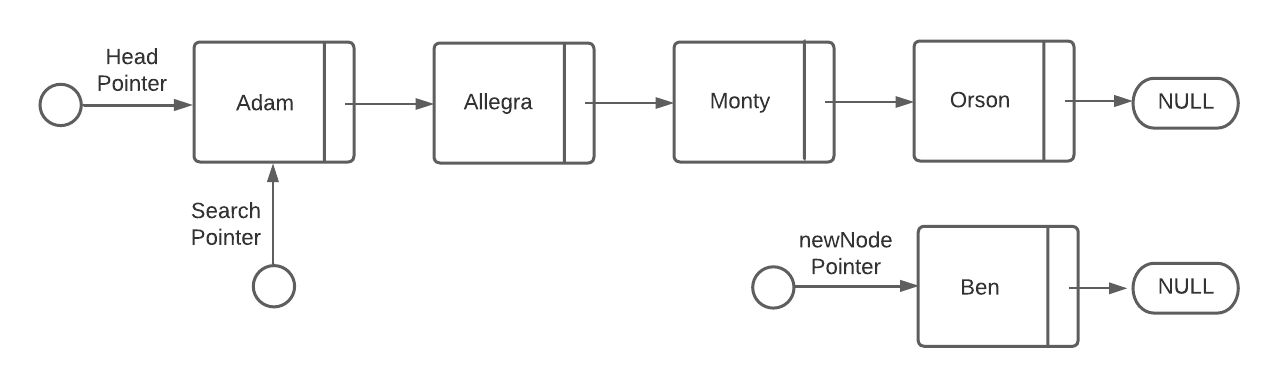With the aid of diagrams, explain how a node containing the name "Ben" would be inserted into the linked list above.
Question 45[1 Mark]
Choose the one option below which is NOT an example of primary memory
(a).
512 GB of Solid State Drive (SDD)
(b).
512 GB of Random-Access Memory (RAM),
(c).
8MB of Level 2 processor cache
(d).
Question 46[3 Marks]
Write an algorithm which outputs every item of a 2D array that is 100 by 100 elements in size
Question 47[3 Marks]
Identify three main functions of an Operating System (OS).
Question 48[4 Marks]
Construct a truth table for the following Boolean expression.
X = not P or Q and P
Question 49[2 Marks]
State the number of different integers that may be represented by 2 bytes of memory and the number of hexadecimal characters that would be required to represented those same 2 bytes.
Question 50[1 Mark]
Which of the following gates implement the equivalent logic to the configuration represented in the diagram below?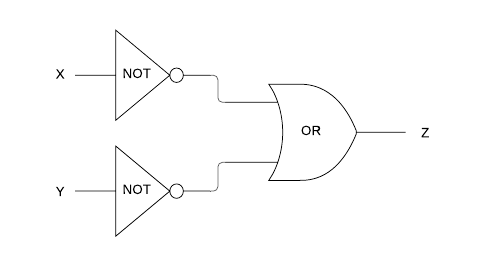(a).
AND
(b).
NOR
(c).
XOR
(d).
NAND
Question 51[1 Mark]
What single gate is logically equivalent to the configuration represented in the diagram below?Question 52[20 Marks]
The Medicare Levy Surcharge (MLS) is a 1-2% levy paid by Australian taxpayers who do not have private hospital cover and who earn above a certain income. The current income threshold is AUD$90,000 for singles and AUD$180,000 for couples / families (2020-2021 financial year). Once Australians earn above these thresholds within a financial year, they must pay the MLS.
(a).
Given an annual income called INCOME, a boolean flag for having private hospital cover or not called PRIVATE and another boolean flag for being single or not called SINGLE, write pseudocode to output True if eligible for the MLS or False otherwise. For example, a single person (SINGLE = true) who doesn’t have private health insurance (PRIVATE = false) and is earning AUD\$120,000 (INCOME = 120000) annually must pay the MLS (output True)

To make the MLS more socially equitable, a range of income tiers determine the relevant MLS percentage to apply to each income. MLS payable is calculated according to the tiers indicated below.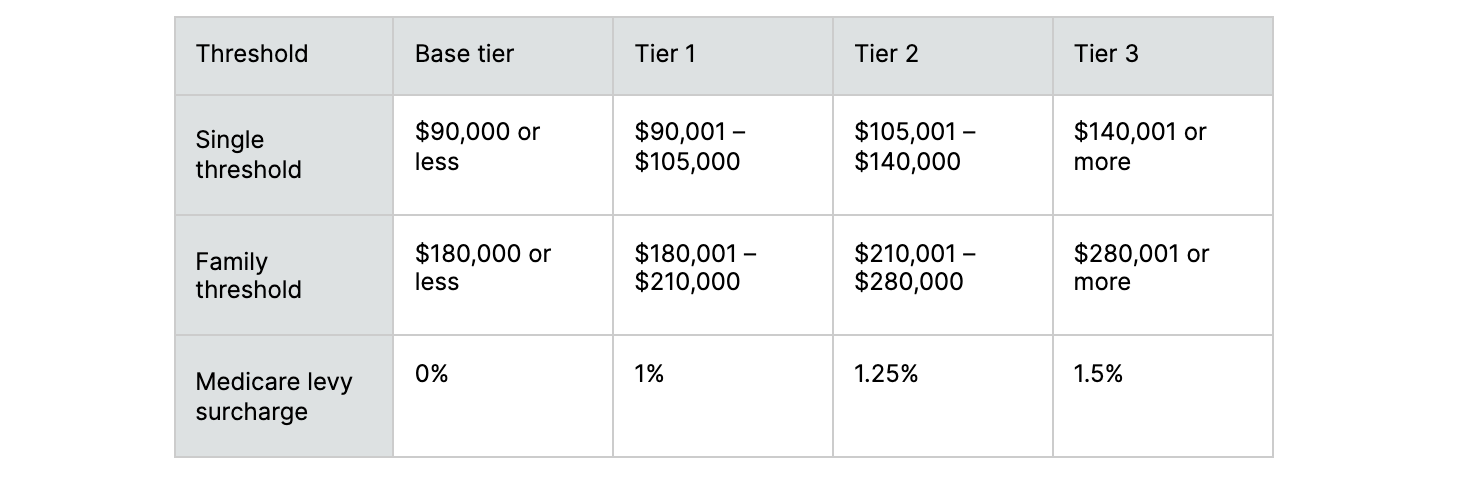(b).
Given the same INCOME parameter described in Part (a) as well as an array called SURCHARGE containing (in ascending order) the MLS percentages for each tier [0, 0.01, 0.0125, 0.015], write pseudocode to determine the amount of MLS dollars owing for a single person who doesn’t have private health insurance in the 2020-2021 financial year.

To improve both code maintainability and extensibility, hard coded tier threshold numbers will instead reference a 2D array called MLS_THRESHOLD which is defined below. For example, MLS_THRESHOLD provides the number 140000.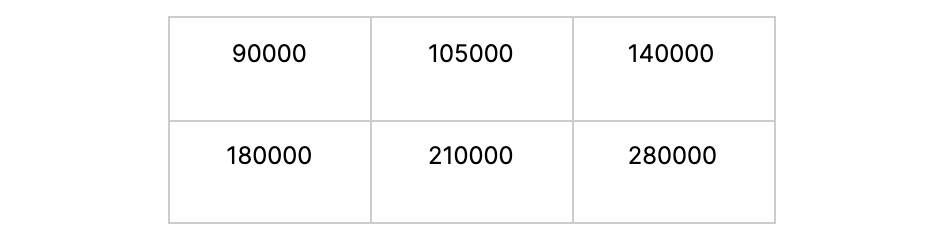(c).
Given the same INCOME and SINGLE parameters described in Part (a) as well as both the SURCHARGE data described in Part (b) and MLS_THRESHOLD data above, extend your solution for Part (b) to more generally determine the amount of MLS dollars owing for anybody (both single and non-single people) who do not have private health insurance.

Question 53[5 Marks]
Consider the following algorithm.
function fun(N) if N = 0 then return 1 else if (N % 2 == 0) return N - fun(N-1) else return N + 2 × fun(N-1) end if end function
(a).
State what would occur if the first "if statement" were removed.

(b).
Trace the algorithm when N=4 by completing the following trace table.

N | fun(N) returns ------------------- 4 | 3 | 2 | 1 | 0 |
(c).
State the final output of the algorithm when N=4.

Question 54[4 Marks]
function f(N) if (N = 0) then return 0 else return N + f(N - 1) end if end function
(a).
With respect to the recursive function above called with N=6, complete the trace table below.

N | f(N) returns ----------------- 6 | 5 | 4 | 3 | 2 | 1 | 0 |
(b).
State the final output when N=7.

Question 55[1 Mark]
if NOT X AND Y then output TRUE else output FALSE end if
With respect to the pseudocode above and trace table below, choose the correct boolean values for outputs N & M.
+-------+-------+----------+ | X | Y | OUTPUT | +-------+-------+----------+ | FALSE | FALSE | FALSE | | FALSE | TRUE | N | | TRUE | FALSE | M | | TRUE | TRUE | FALSE | +-------+-------+----------+
(a).
N = FALSE and M = FALSE
(b).
N = FALSE and M = TRUE
(c).
N = TRUE and M = FALSE
(d).
N = TRUE and M = TRUE
Question 56[2 Marks]
State how many integers could be represented by 2 bytes and how many hexadecimal characters would be required to represent the same 2 bytes.
Question 57[2 Marks]
Outline the purpose of cache memory.
Question 58[3 Marks]
Identify three resources an Operating System (OS) is responsible for managing.
Question 59[1 Mark]
State how many unique memory addresses can be represented using 9 bits? Provide your answer in the denary (base 10) number system.
Question 60[1 Mark]
State how many different colours can be represented by two hexadecimal characters.
Question 61[2 Marks]
Outline how an Operating System (OS) manages primary memory.
Question 62[1 Mark]
Of the statements, select the valid reason for the using hexadecimal numbers in assembly code.
(a).
Hexadecimal numbers use less space in memory
(b).
Hexadecimal numbers are more quickly processed by the CPU
(c).
(d).
Hexadecimal numbers are easier for humans to work with
Question 63[2 Marks]
Outline the function of the data bus in the machine execution cycle.
Question 64[1 Mark]
Convert the binary number 01011100 to hexadecimal (base 16)
(a).
0512
(b).
5C
(c).
5B
(d).
1130
(e).
92
Question 65[1 Mark]
Calculate the largest memory address that can be represented using 9 bits. Provide your answer in the denary (base 10) number system.
Question 66[4 Marks]
Construct an algorithm using an empty stack called STACK to reverse an array containing 7 elements called ARRAY.
Question 67[3 Marks]
Contrast the use of a linked list with an array for capturing streaming measurements from a weather station hygrometer (a device which measures humidity).
Question 68[1 Mark]
Identify which of the following statements relating to static and/or dynamic data structures is false.
(a).
More space is required to keep N values in a static array of length N than in dynamic data structures such as binary trees.
(b).
Random access is a characteristic typical of static arrays.
(c).
It is typically more efficient to maintain an ordered set of values when inserting new elements into dynamic data structures such as linked-lists rather than into static data structures such as arrays.
(d).
Dynamic data structures such as linked-lists typically allocate space more efficiently than static data structures such as arrays.
Question 69[2 Marks]
Outline how binary tree traversal relates to recursion.
Question 70[1 Mark]
A search algorithm uses recursion to sort a one-dimensional array. On each recursive step the algorithm applies one pass of selection sort to have the last element in the array in its correctly ordered position then returns the concatenation of the next recursive call result together with the last element. The next recursive call is provided a sub array where the last element of the array has been removed. The above logic is captured in the following pseudocode.
function recSort(ARRAY) // base case required END_SORTED_ARRAY = onePassSelectionSort(ARRAY) return recSort(subArray(END_SORTED_ARRAY, 0, ARRAY.length - 2)) + END_SORTED_ARRAY[ARRAY.length - 1] end function
Identify, in words or pseudocode, the base case condition of this sorting algorithm.
Question 71[5 Marks]
Describe a recursive function called reverse(buffin, buffout, hay) which moves values from a queue called buffin into another queue called buffout. Use an intermediate stack called hay such that resultant numbers in buffout are, with respect to their original sequence in BUFFIN, in reverse order. Both buffout and hay are initially empty.
Question 72[2 Marks]
With reference to abstraction, outline the utility of operating systems
Question 73[1 Mark]
Which of the following is the biggest number?
(a).
11010101 (binary - base 2)
(b).
(c).
(d).
192 (denary - base 10)
Question 74[1 Mark]
Of the following items, select the one which is not stored in RAM
(a).
(b).
Instructions
(c).
Clock cycles
(d).
Numeric values
Question 75[1 Mark]
Determine the hexadecimal value of the following binary expression 10 1011 1010 1101
Question 76[3 Marks]
Outline when and why Virtual Memory (VM) is used.
Question 77[1 Mark]
If one ASCII character requires 8 bits of memory, how many characters can be stored in 4 bytes?
(a).
4 characters
(b).
8 characters
(c).
12 characters
(d).
2 characters
Question 78[2 Marks]
Outline the primary function of the Memory Address Register (MAR) in the machine execution cycle.
Question 79[2 Marks]
Outline the primary function of the Memory Data Register (MDR) in the machine execution cycle.
Question 80[1 Mark]
Which of the following memory components is not typically found in the CPU?
(a).
(b).
Mode Register (MR)
(c).
Memory Data Register (MDR)
(d).
L2 cache
Question 81[1 Mark]
In relation to the machine execution cycle, state the primary function of the Control Unit (CU).
Question 82[1 Mark]
State the primary function of the ALU in a CPU or GPU.
Question 83[12 Marks]
The following table represents a 2D array called SEMISORTED where each row contains elements sorted in ascending order. The rows are not sorted in relation to each other.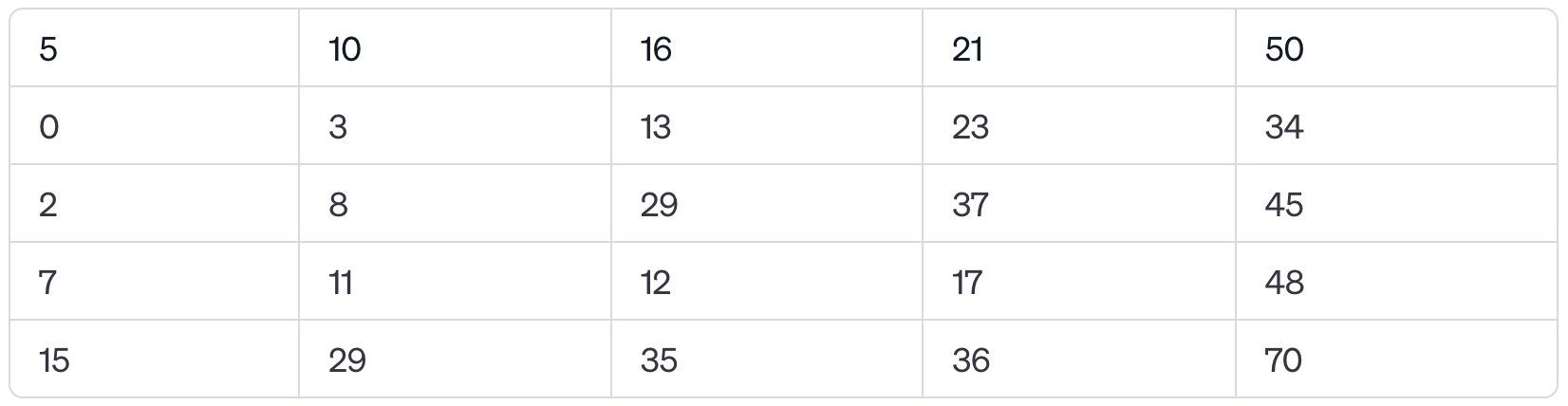(a).
State the value of SEMISORTED

(b).
Construct an algorithm that outputs every element in the 2D array SEMISORTED.

(c).
Modify the algorithm in b) to efficiently search the 2D array SEMISORTED for a given number K. If K is present output TRUE, otherwise output FALSE.

(d).
Suggest how the 2D array SEMISORTED might be more efficiently searched using a combination of linear and binary search. Also indicate the characteristic of the 2D array SEMISORTED data that makes this optimisation convenient.

Question 84[1 Mark]
Often the same function may be coded either recursively or iteratively. Which of the following is NOT a typical advantage of recursion over iteration?
(a).
Trees data structures are more easily traversed.
(b).
Code is more succinct.
(c).
Functions use less memory.
(d).
Fibonacci sequences are more easily produced.
Question 85[10 Marks]
(a).
Determine the decimal value of the binary ‘1001’

(b).
Determine the binary value of the denary ‘67’

(c).
Outline why the binary number system is used in computing

To convert decimal to binary, we use a simple handwritten algorithm, this same logic can be applied in pseudocode.
(d).
In pseudocode, using a stack, write an algorithm that finds the binary value of a decimal input by outputting the digits one by one

Question 86[1 Mark]
State why the hexadecimal number system is frequently used in computing.
Question 87[2 Marks]
One of the functions of an operating system is memory management. Describe how this function prevents the system from crashing when more than one program is run at the same time.
Question 88[14 Marks]
The collection HUMIDITY contains humidity readings recorded for the city of Melbourne over the course of one working week (5 days), starting on Monday and ending on Friday (inclusive). Each day, 24 readings were taken - one each hour. Each day the first reading is taken at 00:00, the second at 01:00 and so on through until 23:00. The data is stored in a collection in chronological order with the data for Monday stored in the first node followed by Tuesday and so on.
(a).
State the total number of readings that were taken during this week.

(b).
Construct an algorithm to read the HUMIDITY data into a 2D array called MEASUREMENTS.

(c).
Contrast one advantage of having data in this new D array MEASUREMENTS data structure over the original HUMIDITY collection

(d).
Using the 2D MEASUREMENTS array from part (b), construct an algorithm to identify when the LOWEST humidity reading was recorded in a given week. Output both the day and the reading number for that day when the LOWEST reading occurred (for example, the 3rd reading on the 4th day of readings would be described as "Thursday 3"). You are also given a 1D array called NAMES which contains text strings starting from Monday through to Sunday sequentially (for example NAMES yields the string 'Wednesday').

Question 89[1 Mark]
A binary tree is made from the following ordered set {9, 13, 4, 7, 5, 18, 2}, which of the following statements is true about the tree?
(a).
13 is a leaf node
(b).
7 is at a greater depth than 2 in the tree
(c).
7 is a leaf node
(d).
5 is at a greater depth than 18 in the tree
Question 90[4 Marks]
An experimental new laptop is being developed with 512gb of primary memory and no persistent storage beyond a small amount reserved for the Operating System only (insisting users use cloud storage for their files)
Outline a positives and negative of this design choice
Question 91[1 Mark]
State why the hexadecimal number system is frequently used in computing.
Question 92[2 Marks]
One of the functions of an operating system is memory management. Describe how this function prevents the system from crashing when more than one program is run at the same time.
Question 93[3 Marks]
Explain the characteristics of cache memory
Question 94[3 Marks]
Describe the key characteristics and types of primary memory
Question 95[1 Mark]
Explain why the vast majority of computers are based on the binary number system.
Question 96[2 Marks]
Question 97[2 Marks]
Outline the relationship between binary and hexadecimal.
Question 98[3 Marks]
Explain why deleting the first node in this list is different to deleting other nodes.
Question 99[4 Marks]
Create a truth table for the following expression: A and B or not C
Question 100[1 Mark]
Define the phrase 'operating system.'
Question 101[4 Marks]
Consider those algorithms which apply the principle of divide and conquer to more efficiently implement search.
(a).
State the name of a dynamic data structure suitable for maintaining a list of names in such a way as to facilitate faster searching.

(b).
Sketch the data structure suggested in part (a) formed as the following names are provided sequentially from left to right, that is, Kris is input first and Linda last.

Kris, Andrew, Dan, Kate, Linda
Question 102[4 Marks]
Outline the steps of the Machine Instruction Cycle
Question 103[3 Marks]
Outline, with the aid of an example, why many widely used applications do not come preinstalled on operating systems
Question 104[1 Mark]
How many hexadecimal characters would be required to represent 2 bytes.
(a).
1
(b).
4
(c).
8
(d).
16
Question 105[6 Marks]
Construct pseudocode to populate a 3x4 (rows x columns) 2D array called FILTERED with as much data from a stack called NUMBERS as will fit and not attempt to write outside the bounds of the array. Fill FILTERED starting from position [0,0] and sequentially fill rows first (with no gaps between elements). Include in FILTERED only those numbers from NUMBERS which are divisible by 7.
Question 106[2 Marks]
Explain why cache memory can speed up the processing within a computer.
Question 107[10 Marks]
A search algorithm uses recursion to sort a one-dimensional array by sorting the end of the array and returning the array minus the last element at each recursive step.
(a).
Identify, in words, what the base case of this sorting algorithm must be

(b).
Outline how binary tree traversal uses the concept of recursion

Consider the following algorithm func(N) if N=0 then return 1 else if (N % 2 == 0) return N - func(N-1) else return N + func(N-1) end if end method
(c).
State what would occur if the first "if statement" was removed

(d).
Trace the algorithm when N=4

(e).
State the final output of the algorithm when N=4

Question 108[5 Marks]
Construct pseudocode to populate a queue called DELAYED from a 5x7 (rows x columns) 2D array called TIMES. Fill DELAYED by extracting values starting from position [0,0] in the TIMES array by sequentially extracting data from an entire row before moving to the next row. Add to those extracted TIMES a delay value of 3 as they are moved to the DELAYED queue.
Question 109[1 Mark]
Outline the functions of the ALU.
Question 110[1 Mark]
Outline the primary function of an ALU in a CPU or GPU.
Question 111[3 Marks]
Explain the characteristics of cache memory.
Question 112[3 Marks]
Describe the key characteristics and types of primary memory

HomeThe Right Test - Fast